# 3. Let f: R+R be a function. (a) Assume that f is Riemann integrable on [a,...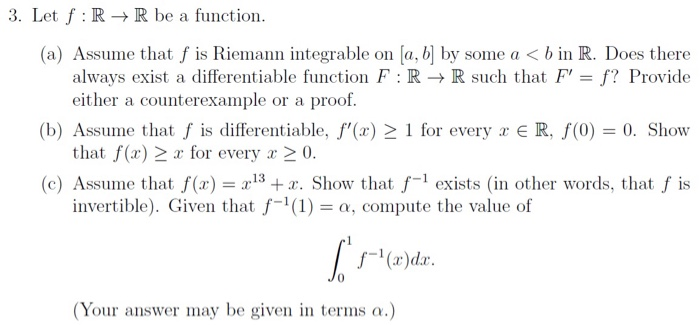3. Let f: R+R be a function. (a) Assume that f is Riemann integrable on [a, b] by some a < b in R. Does there always exist a differentiable function F:RR such that F' = f? Provide either a counterexample or a proof. (b) Assume that f is differentiable, f'(x) > 1 for every x ER, f(0) = 0. Show that f(x) > x for every x > 0. (c) Assume that f(x) = 2:13 + x. Show that f-1 exists in other words, that f is invertible). Given that f-l(1) = a, compute the value of [ s-'(x)dır. (Your answer may be given in terms a.)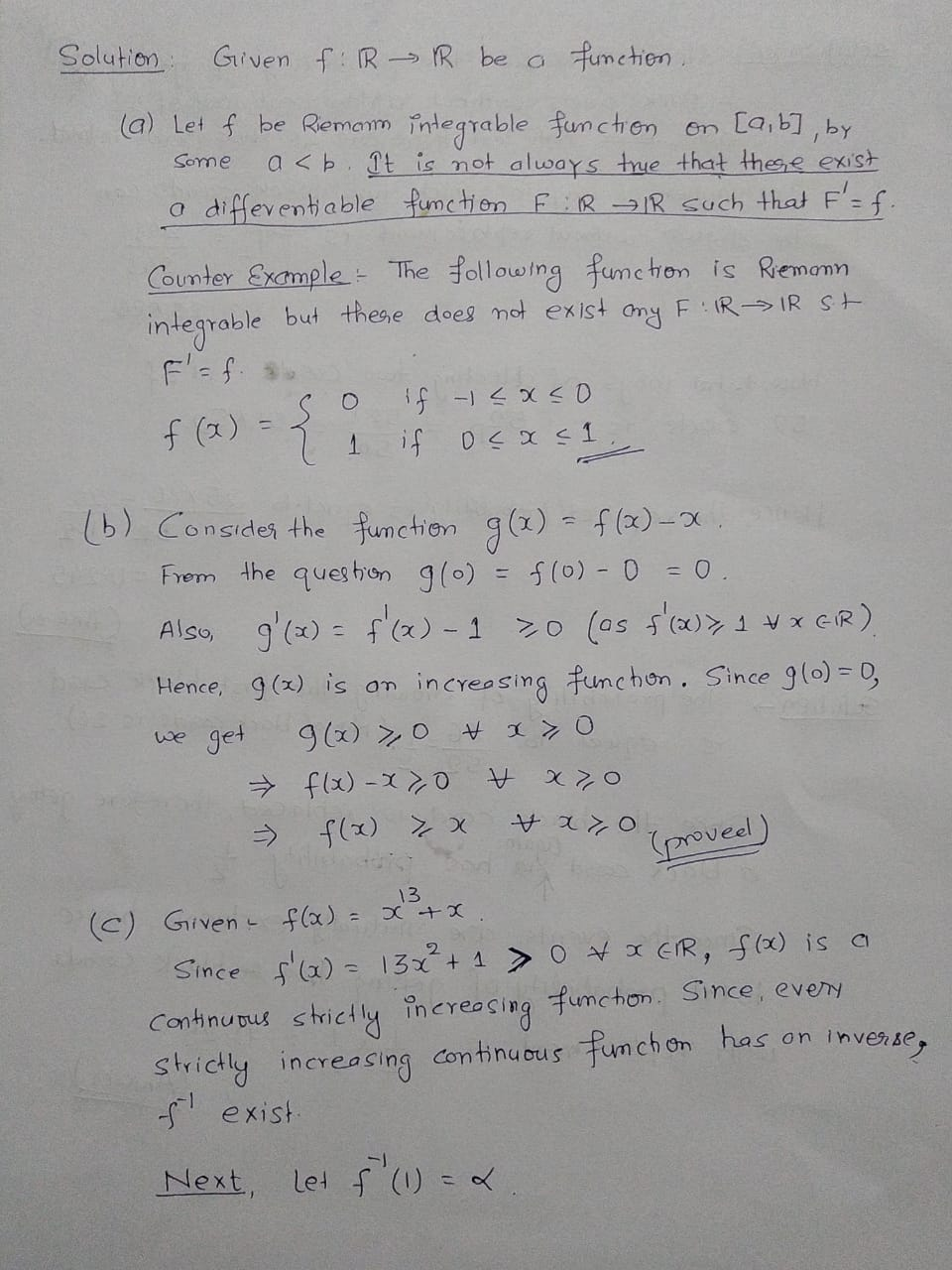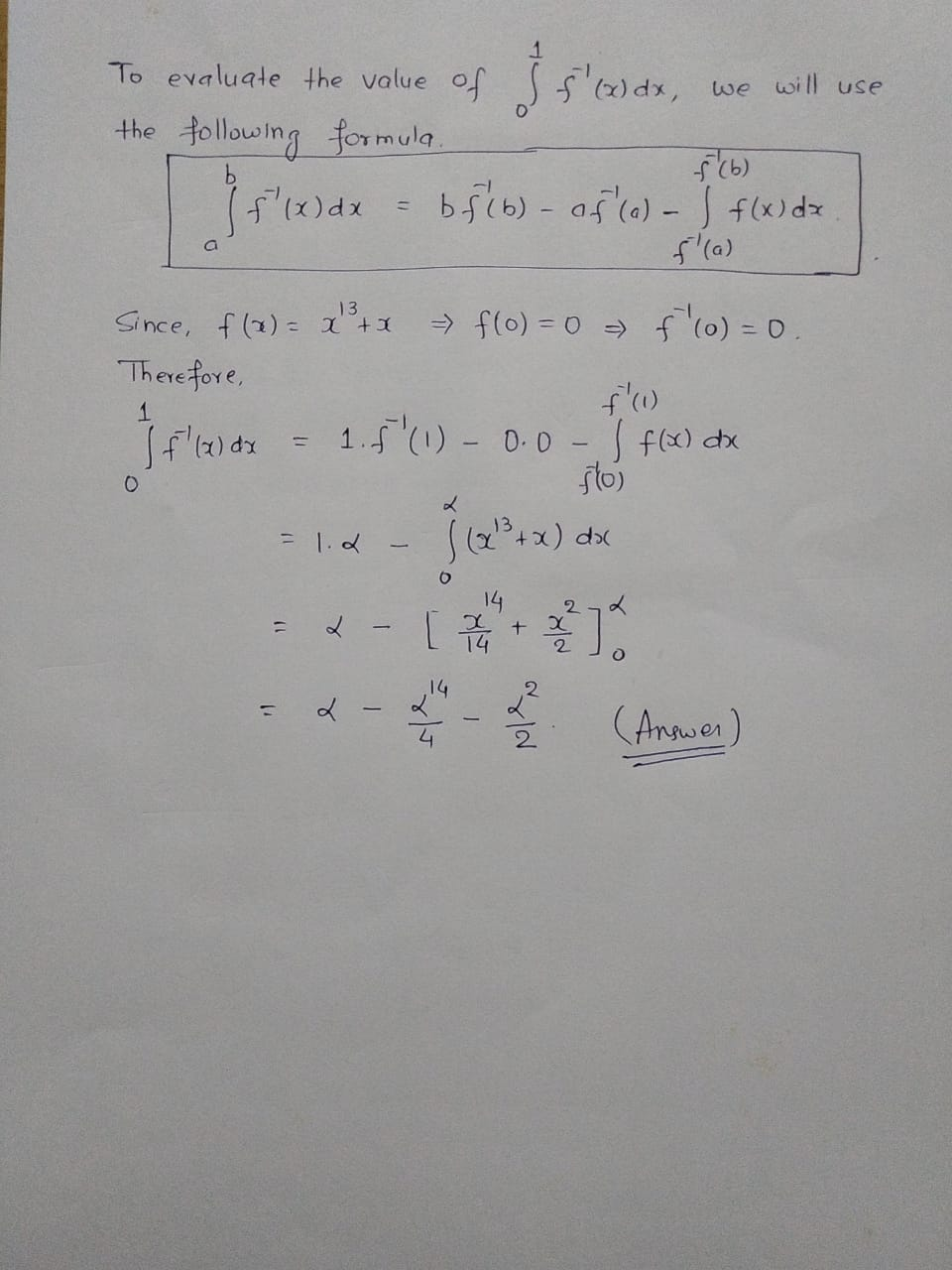##### Add Answer of: 3. Let f: R+R be a function. (a) Assume that f is Riemann integrable on [a,...
Similar Homework Help Questions
• ### Problem 1. Consider the function f(x)- 3.12 show that f is Riemann integrable on [0.2] and use the definition to f...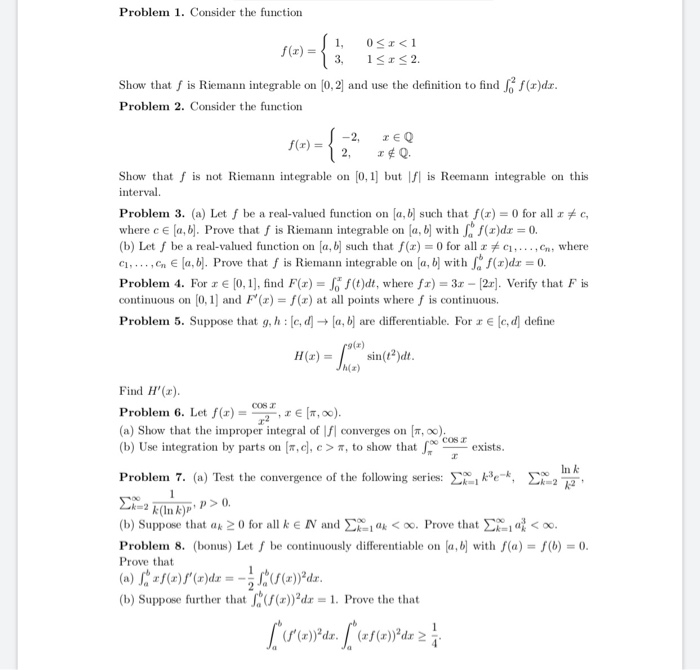Problem 1. Consider the function f(x)- 3.12 show that f is Riemann integrable on [0.2] and use the definition to find .后f(x)dr Problem 2. Consider the function -2, zEQ 2, O f(r) = Show that f is not Riemann integrable on 0,1 but s Reemann integrable on this interval. Problem 3. (a) Let f be a real-valued function on a, b] such thatf()0 for all c, where c E [a, b Prove that f is Riemann integrable on a, b...

• ### 4. (a) Assume a function h is differentiable at some point to. Is it true that...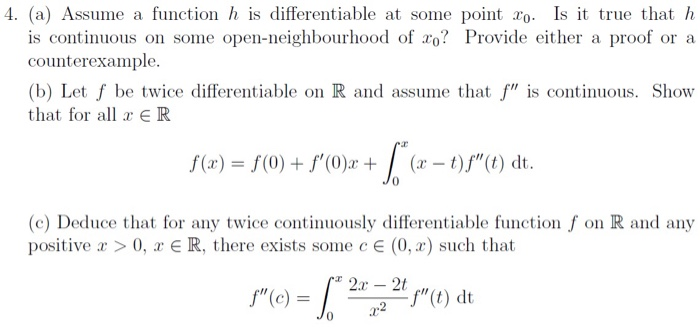4. (a) Assume a function h is differentiable at some point to. Is it true that h is continuous on some open-neighbourhood of xo? Provide either a proof or a counterexample. (b) Let f be twice differentiable on R and assume that f" is continuous. Show that for all x ER S(x) = S(0) + s°C)x + [ (x - 1))"(dt. (C) Deduce that for any twice continuously differentiable function f on R and any positive x > 0, x...

• ### 6. The goal of this problem is to prove that a function is Riemann integrable if and only if its ...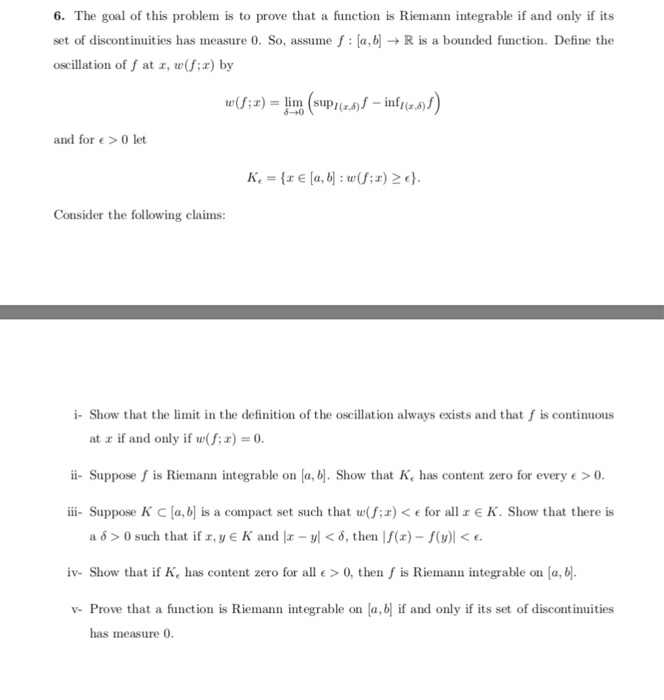exercice 6 6. The goal of this problem is to prove that a function is Riemann integrable if and only if its set of discontinuities has measure 0. So, assume f: a, bR is a bounded function. Define the oscillation of f at , w(f:z) by and for e >0 let Consider the following claims: i- Show that the limit in the definition of the oscillation always exists and that f is continuous at a if and only if w(f;...

• ### Exercise 3. Let f : [0,1]- R be non-negative and Riemann integrable. Assume of()dr 0. otherwise. Show that g is not Rie...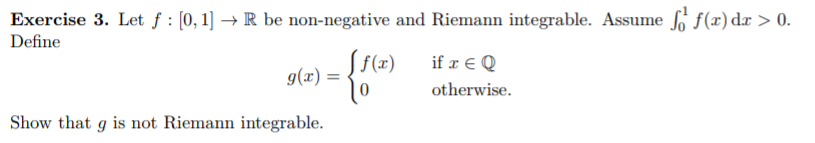Exercise 3. Let f : [0,1]- R be non-negative and Riemann integrable. Assume of()dr 0. otherwise. Show that g is not Riemann integrable Exercise 3. Let f : [0,1]- R be non-negative and Riemann integrable. Assume of()dr 0. otherwise. Show that g is not Riemann integrable

• ### 1. (20 pts) Let f : [a, b] R and xo € (a,b). Assume that f...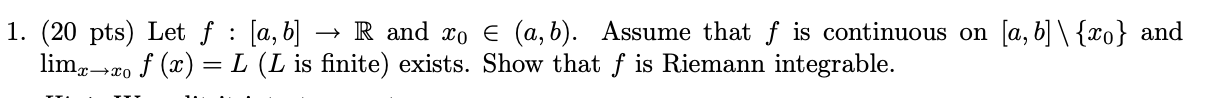1. (20 pts) Let f : [a, b] R and xo € (a,b). Assume that f is continuous on [a, b] \ {xo} and lim, L (L is finite) exists. Show that f is Riemann integrable. 2x=20 f (x)

• ### Prove Riemann Integrable -- updatedLet f be a Riemann Integrable function defined on [a,b] and x0Є[a,b]. Show that if g is a bounded function that satisfies g(x)=f(x) forxЄ[a,b]{x0}, then g is Riemann integrable andOk so I have gotten through so of this proof:g(x) = f(x) for x contained in [a,b] but not {x0} implies that g(x) =f(x) for x in [a, x0-1/n] and g(x) = f(x) for x in[x0+1/n, b] assuming n is large.ThereforeNow consider sincef is Riemann Integrable and n is large. Using...

• ### Riemann integrable

If f:[a,b]->R is a bounded function such that f(x)=0 except for x E {c1,c2,...cn} C [a,b], show that f is Riemann integrable on [a,b] and determine the Riemannintegral value.

• ### Problem 5 (4 points) Suppose f : (0,1] → R is Riemann integrable on [c, i] for every c> 0. Define 1 c→0 if the limit erists and is finite. If f is (even) Riemann integrable on [0, 1], show that th...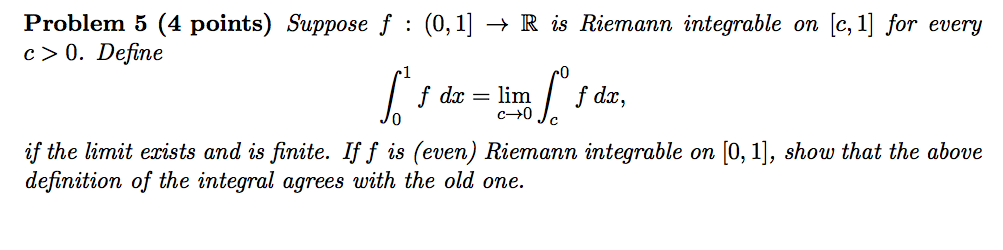Problem 5 (4 points) Suppose f : (0,1] → R is Riemann integrable on [c, i] for every c> 0. Define 1 c→0 if the limit erists and is finite. If f is (even) Riemann integrable on [0, 1], show that the above definition of the integral agrees with the old one. Problem 5 (4 points) Suppose f : (0,1] → R is Riemann integrable on [c, i] for every c> 0. Define 1 c→0 if the limit erists and...

• ### Let f : [a, b] → R and xo e (a,b). Assume that f is continuous...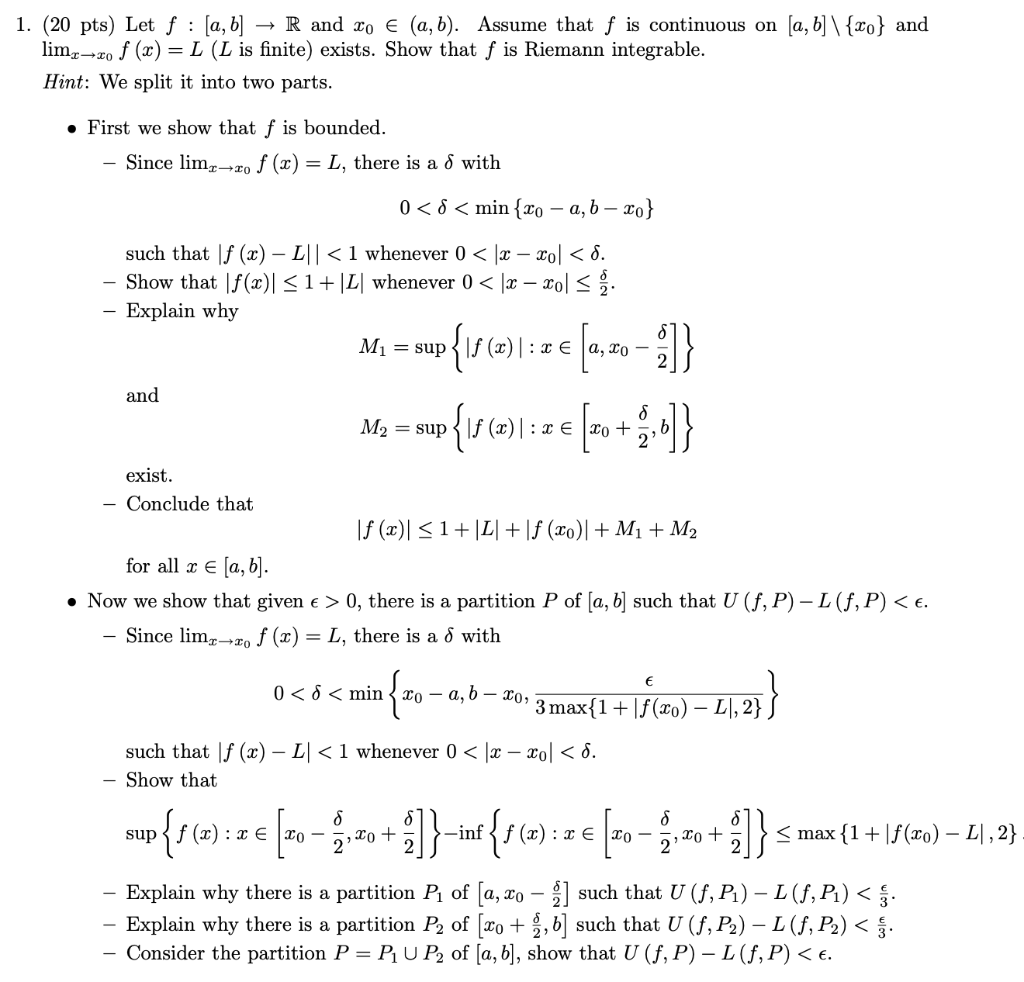Let f : [a, b] → R and xo e (a,b). Assume that f is continuous on [a,b] \{x0} and lim x approaches too x0 f(x) = L (L is finite) exists. Show that f is Riemann integrable. 1. (20 pts) Let f : [a, b] R and to € (a,b). Assume that f is continuous on [a, b]\{ro} and limz-ro f (x) = L (L is finite) exists. Show that f is Riemann integrable. Hint: We split it into...

• ### Riemann integrable

Prove that the function f:[0,1]->R defined by f(0) = 1, f(x) = 0 if x is irrational, and f(m/n)=1/n if m,n E N are relatively prime is Riemann integrable.

Free Homework App